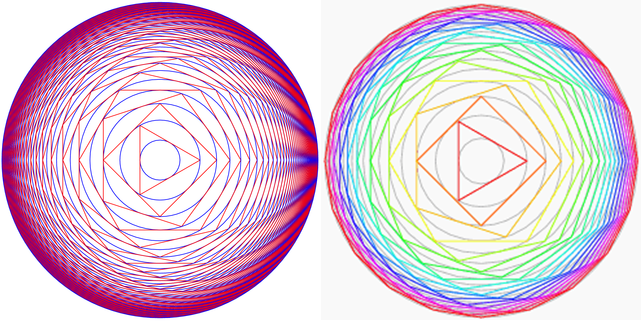# Circumscribed To Infinity

Geometry Level 5Circumscribe a triangle about a unit circle, another circle around the triangle, a square outside this circle, another circle outside the square and so forth.

Let $r$ be the radius of the circle formed when the process is carried forever.

Find the square root of the sum of first 14 decimal places of $r$

You are allowed to compute the answer by calculator or software.

×